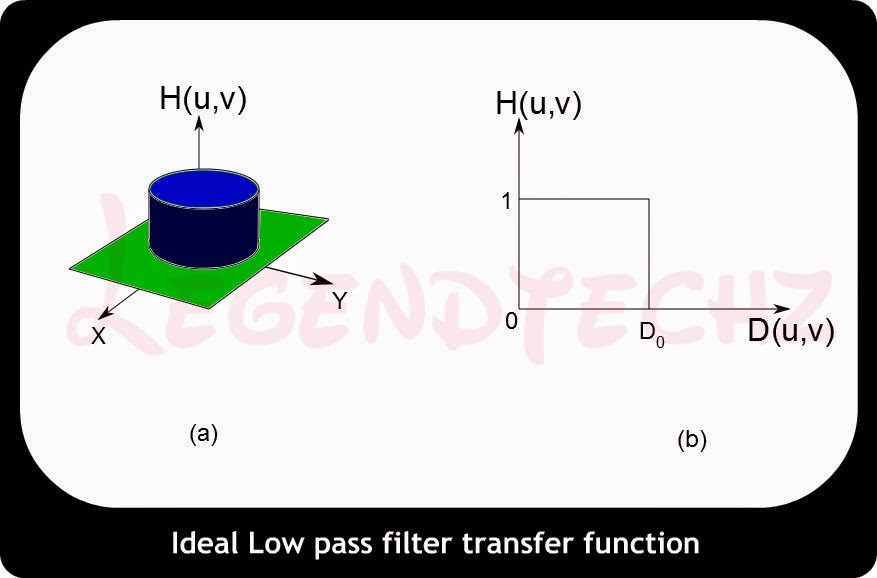35. Explain about Ideal Low Pass Filter (ILPF) in frequency domain.

35. Explain about Ideal Low Pass Filter (ILPF) in frequency domain.

Lowpass Filter:

The edges and other sharp transitions (such as noise) in the gray levels of an image contribute significantly to the high-frequency content of its Fourier transform. Hence blurring (smoothing) is achieved in the frequency domain by attenuating us the transform of a given image.

G (u, v) = H (u, v) F(u, v)

where F (u, v) is the Fourier transform of an image to be smoothed. The problem is to select a filter transfer function H (u, v) that yields G (u, v) by attenuating the high-frequency components of F (u, v). The inverse transform then will yield the desired smoothed image g (x, y).

Ideal Filter:

A 2-D ideal lowpass filter (ILPF) is one whose transfer function satisfies the relation

$H(u,v)=\begin{cases}1 & D(u,v) \leq D_{0}\\0 & D(u,v) > D_{0}\end{cases}$

where D is a specified non negative quantity, and D(u, v) is the distance from point (u, v) to the
origin of the frequency plane; that is,
$D(u,v)=\sqrt{(u^{2}+v^{2})}$
Figure 3 (a) shows a 3-D perspective plot of H (u, v) u a function of u and v. The name ideal filter indicates that oil frequencies inside a circle of radius D0 are passed with no attenuation, whereas all frequencies outside this circle are completely attenuated.Fig.3 (a) Perspective plot of an ideal lowpass filter transfer function; (b) filter cross section.

The lowpass filters are radially symmetric about the origin. For this type of filter, specifying a cross section extending as a function of distance from the origin along a radial line is sufficient, as Fig. 3 (b) shows. The complete filter transfer function can then be generated by rotating the cross section 360 about the origin. Specification of radially symmetric filters centered on the N x N frequency square is based on the assumption that the origin of the Fourier transform has been centered on the square.

For an ideal lowpass filter cross section, the point of transition between H(u, v) = 1 and H(u, v) = 0 is often called the cutoff frequency. In the case of Fig.3 (b), for example, the cutoff frequency is Do. As the cross section is rotated about the origin, the point Do traces a circle giving a locus of cutoff frequencies, all of which are a distance Do from the origin. The cutoff frequency concept is quite useful in specifying filter characteristics. It also serves as a common base for comparing the behavior of different types of filters.

The sharp cutoff frequencies of an ideal lowpass filter cannot be realized with electronic components, although they can certainly be simulated in a computer.